# 系外行星的探测¶

## 径向速度法¶$\begin{split}v_{rad} &= k\left( \cos(\Lambda(t))+e\cos\omega \right) \\ k & = \frac{2\pi a_1\sin i}{P\sqrt{1-e^2}}\end{split}$

$k =C \frac{2\pi m_2 \sin i}{P^{1/3} (m_1+m_2)^{2/3}\sqrt{1-e^2}}.$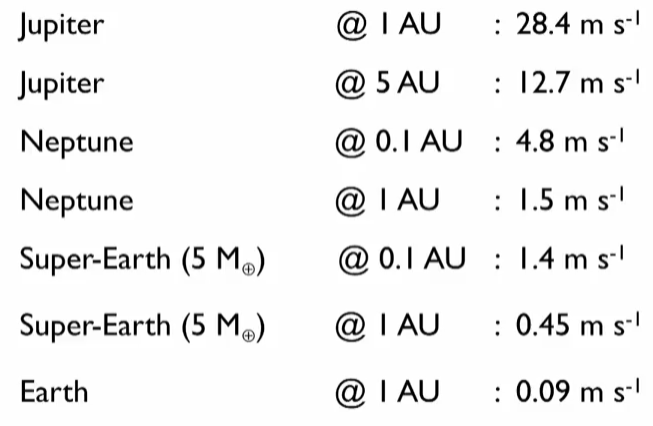HARPS 的光谱测量可以达到 1 m/s 的径向速度测量精度。而这要求仪器能够测量 15 纳米的波长变化，或者说要去能够测量 1/1000 的 CCD 上的单像素上的变化。这样也就需要我们把仪器的温度降低到足够低，使得热涨落降低到不影响我们测量的程度。

## 天体测量学¶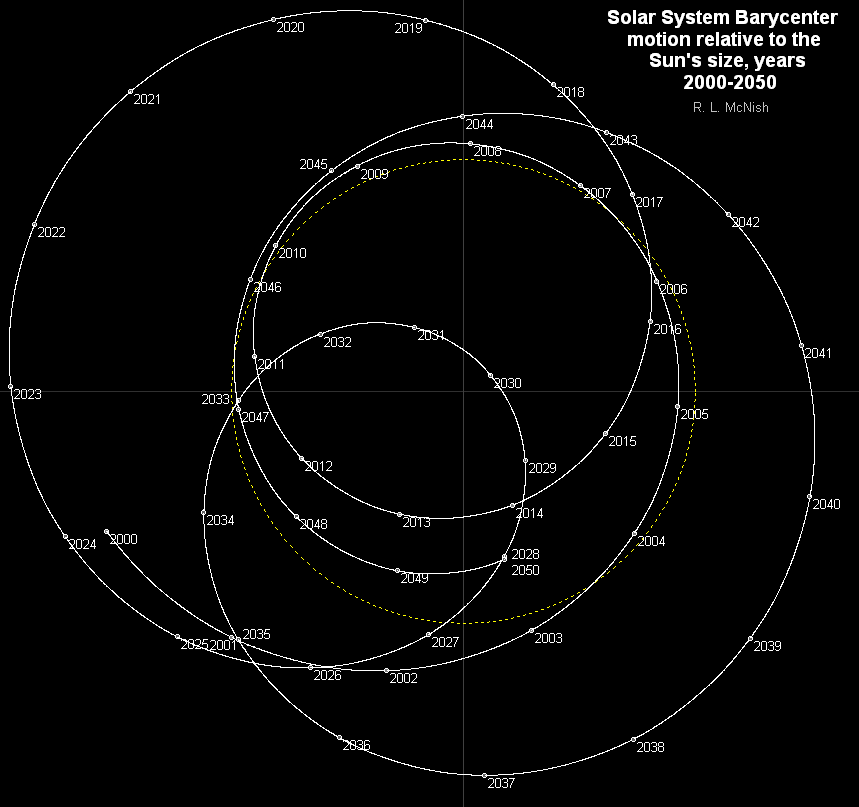$\alpha_1 = \frac{a_1}{d} = \frac{m_2}{d}\left(\frac{P}{m_1+m_2}\right)^{2/3}.$

$m_2 = \alpha_1 d \left(\frac{m_1}{m_2}\right)^{2/3}$

$\alpha_1 = \frac{m_2}{d} \left(\frac{P}{m_1}\right)^{2/3}.$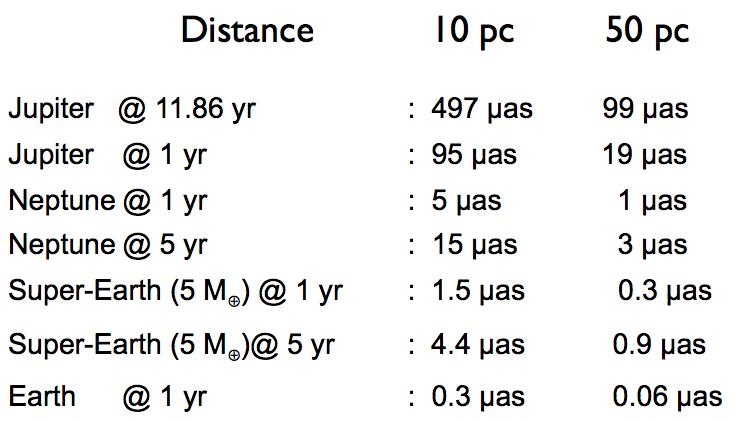ESA 发射了 GAIA 卫星 ，用来精确测量距离并且探测巨行星。以下视频来自 ESA .

## 直接影像¶## 凌日法¶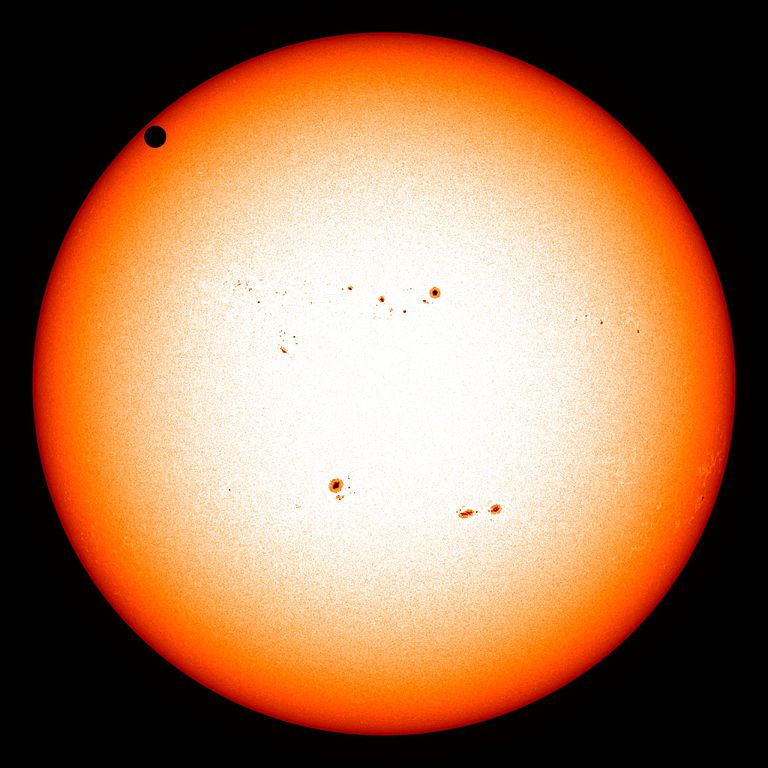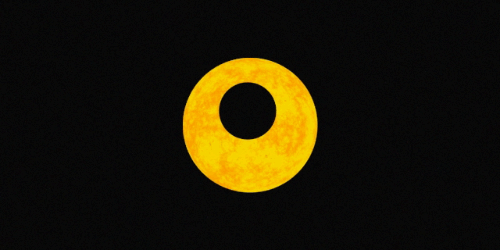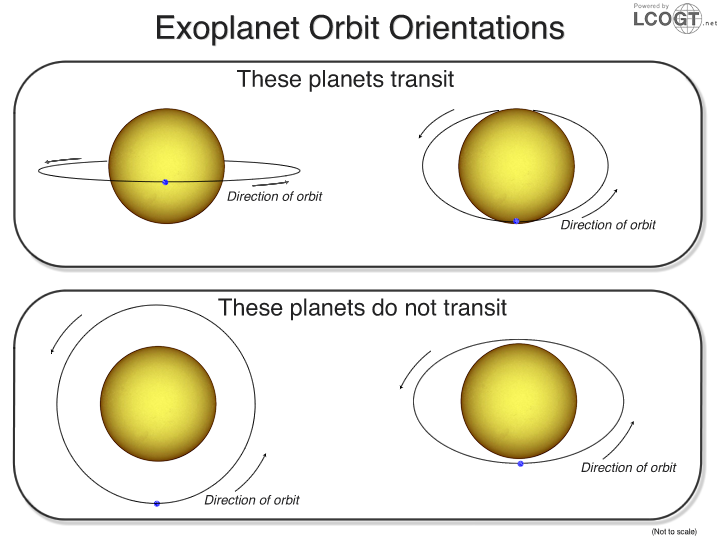1. 行星的大小。行星越大，对恒星的遮挡越大。
2. 恒星的大小。恒星越大，同样大小的行星对它的遮挡越小。
3. 行星距离恒星的距离。行星距离恒星越近，对于一定距离外的观测者，观测到的可能性越大。
4. 行星的周期。行星的周期太长，我们可能不能等到完成一个周期的观测。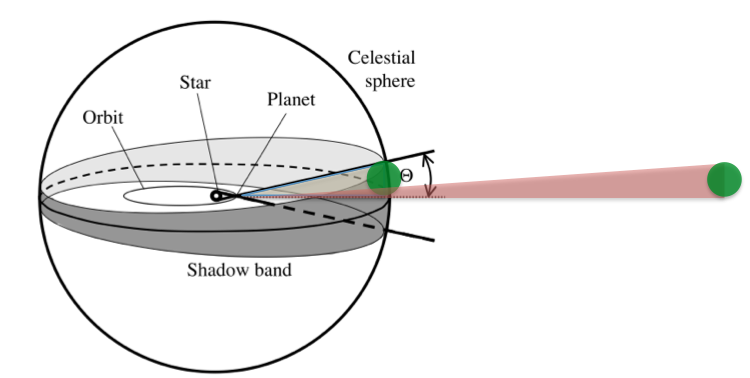$P_{tra}= \left(\frac{R_{S}\pm R_p}{a}\right) \left( \frac{1+e \sin\omega}{1-e^2} \right)$

### 一般讨论¶$f(t) = F(t)/F_S$

1. 行星的反射光

考虑到行星也会反射光，所以不同相位的行星会对总的通量有个影响，会形成一个类似正弦曲线的曲线。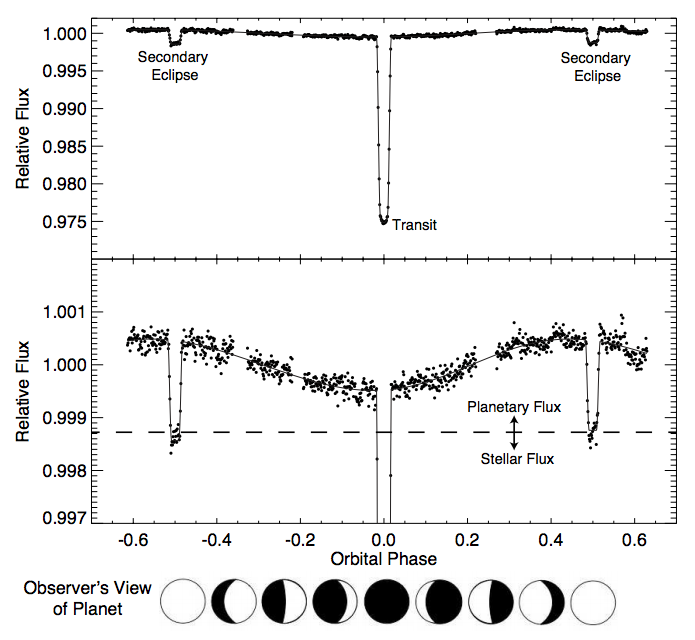2. 观测的波长

3. 恒星并不是一个圆盘，而是一个流动的圆球。

### 计算行星系统参数¶

1. 行星的密度
2. 行星的轨道的参数，包括半长轴，偏心率，周期

1. 除去行星的凌日过程和隐藏（occultation）时间

$\Delta t_c \approx \frac{P}{2}\left( 1+\frac{4}{\pi}e\cos\omega \right)$
2. 行星的凌日时间和隐藏时间的比值

$\frac{T_{occ}}{T_{tra}}\approx \frac{1+e\sin\omega}{1-e\sin\omega}$

### 如何确认一颗行星¶

1. 双星系统，transiting stellar object
2. 背景中可能的星蚀
3. 跟一颗恒星在光学上相隔很近的一个行星系统发生凌日

### 实际观测¶

1. SUPERWASP 口径很小，只有 11 厘米，目的并不是获得足够清晰的图像，而是获取光变曲线，所以并不需要大口径的望远镜。
2. KEPLER 口径要大得多。Kepler 可以获得比较小的行星的光变曲线。而且 Kepler 的观测周期已经有 5 年了，所以它获取的数据可以进行自洽性确认，并且可以观测到较长的周期的行星。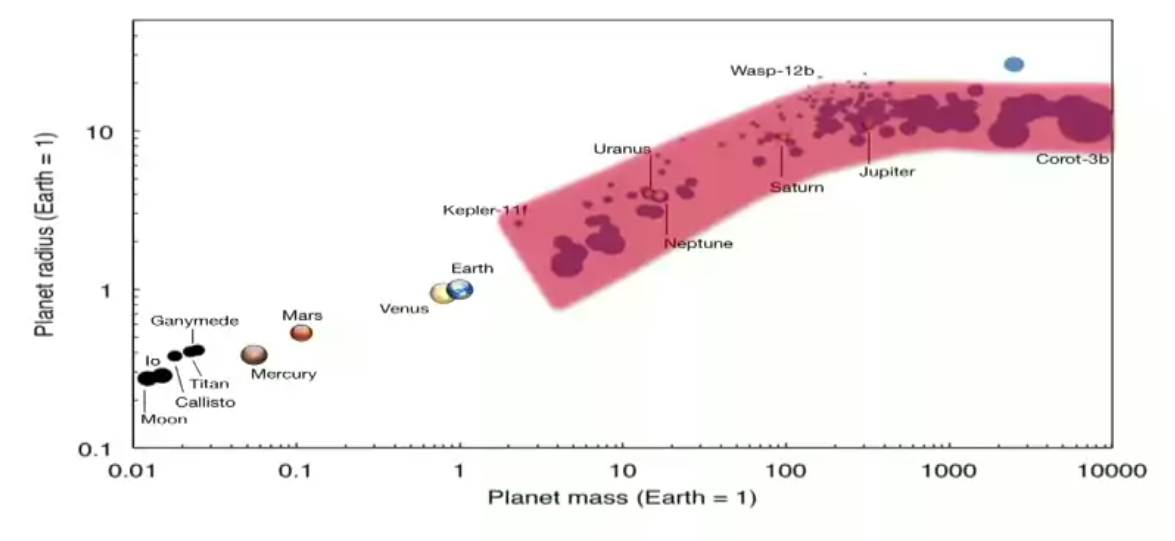## 微引力透镜¶$\theta=\frac{4GM}{rc^2},$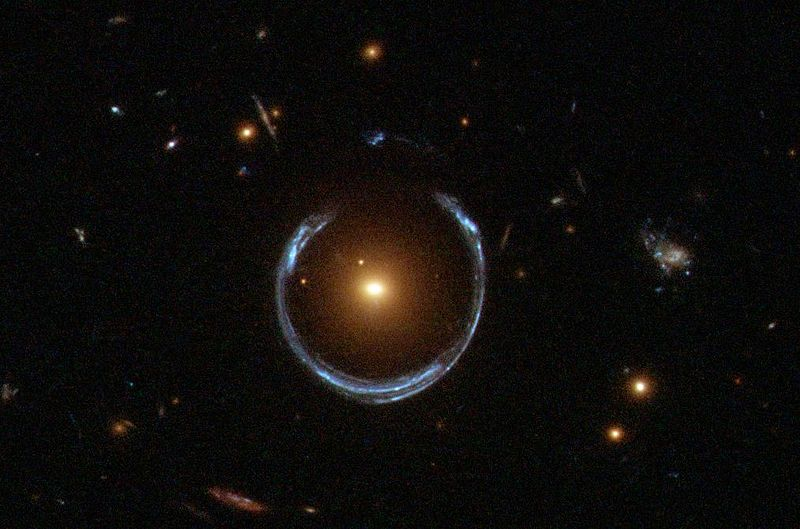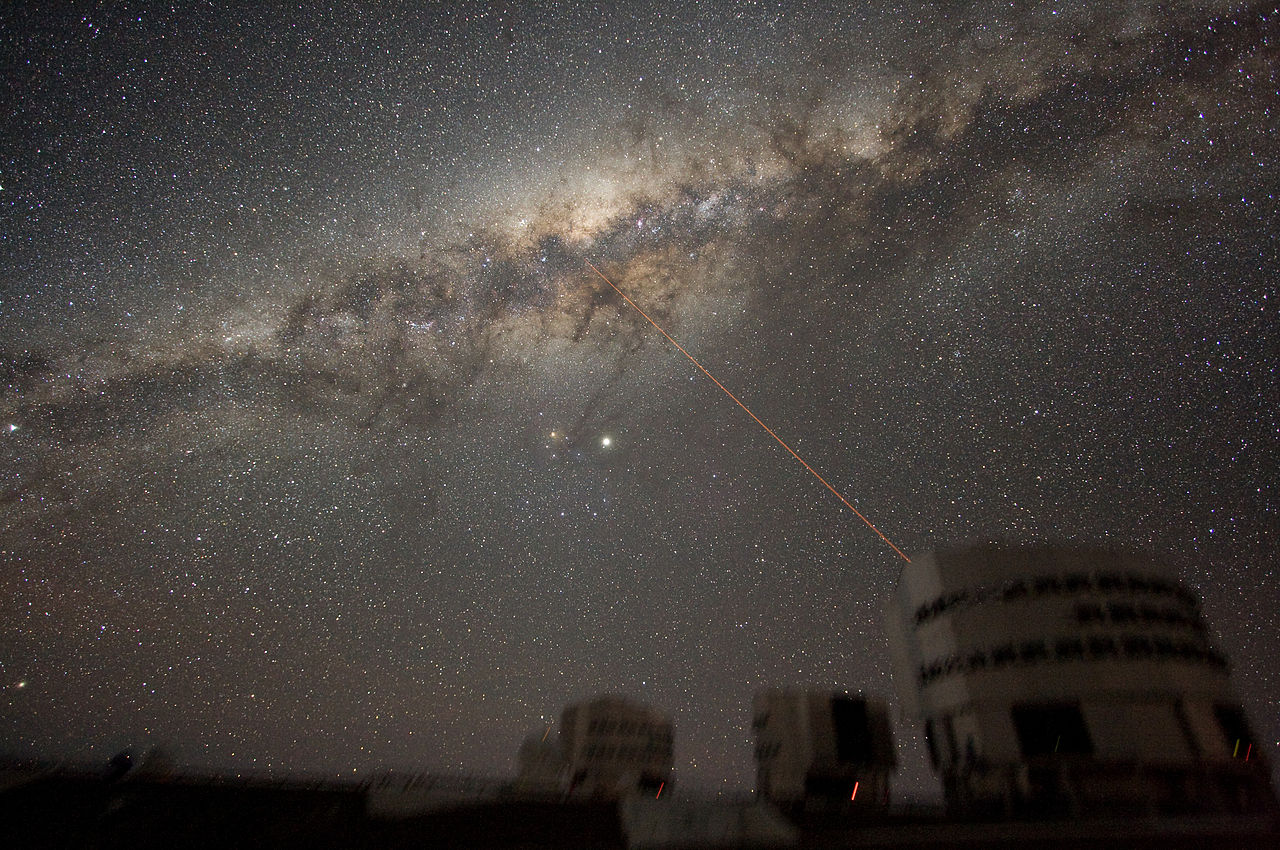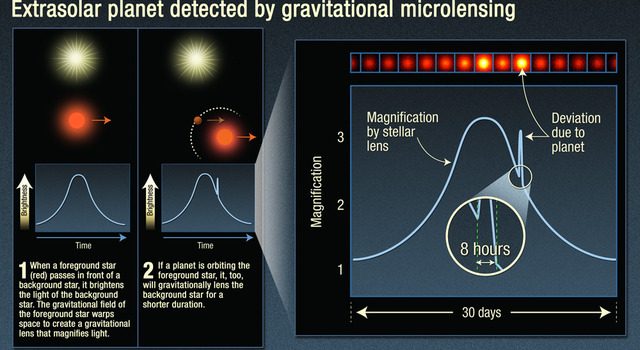## 参考及尾注¶

  (1, 2) 来自 Coursera 的 The Diversities of Exoplanets
  在 Coursera 的 The Diversities of Exoplanets 的第三周的课程中，有一个很生动的演示。
  Rossiter MacLaughlin effect.